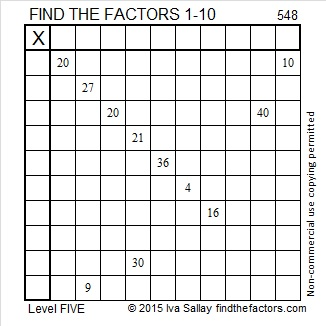# 548 and Level 5

548 is the hypotenuse of the Pythagorean triple 352-420-548. What is the greatest common factor of those three numbers?Print the puzzles or type the solution on this excel file: 10 Factors 2015-07-06

—————————————————————————————————

• 548 is a composite number.
• Prime factorization: 548 = 2 x 2 x 137, which can be written 548 = (2^2) x 137
• The exponents in the prime factorization are 2 and 1. Adding one to each and multiplying we get (2 + 1)(1 + 1) = 3 x 2  = 6. Therefore 548 has exactly 6 factors.
• Factors of 548: 1, 2, 4, 137, 274, 548
• Factor pairs: 548 = 1 x 548, 2 x 274, or 4 x 137
• Taking the factor pair with the largest square number factor, we get √548 = (√4)(√137) = 2√137 ≈ 23.4093998—————————————————————————————————